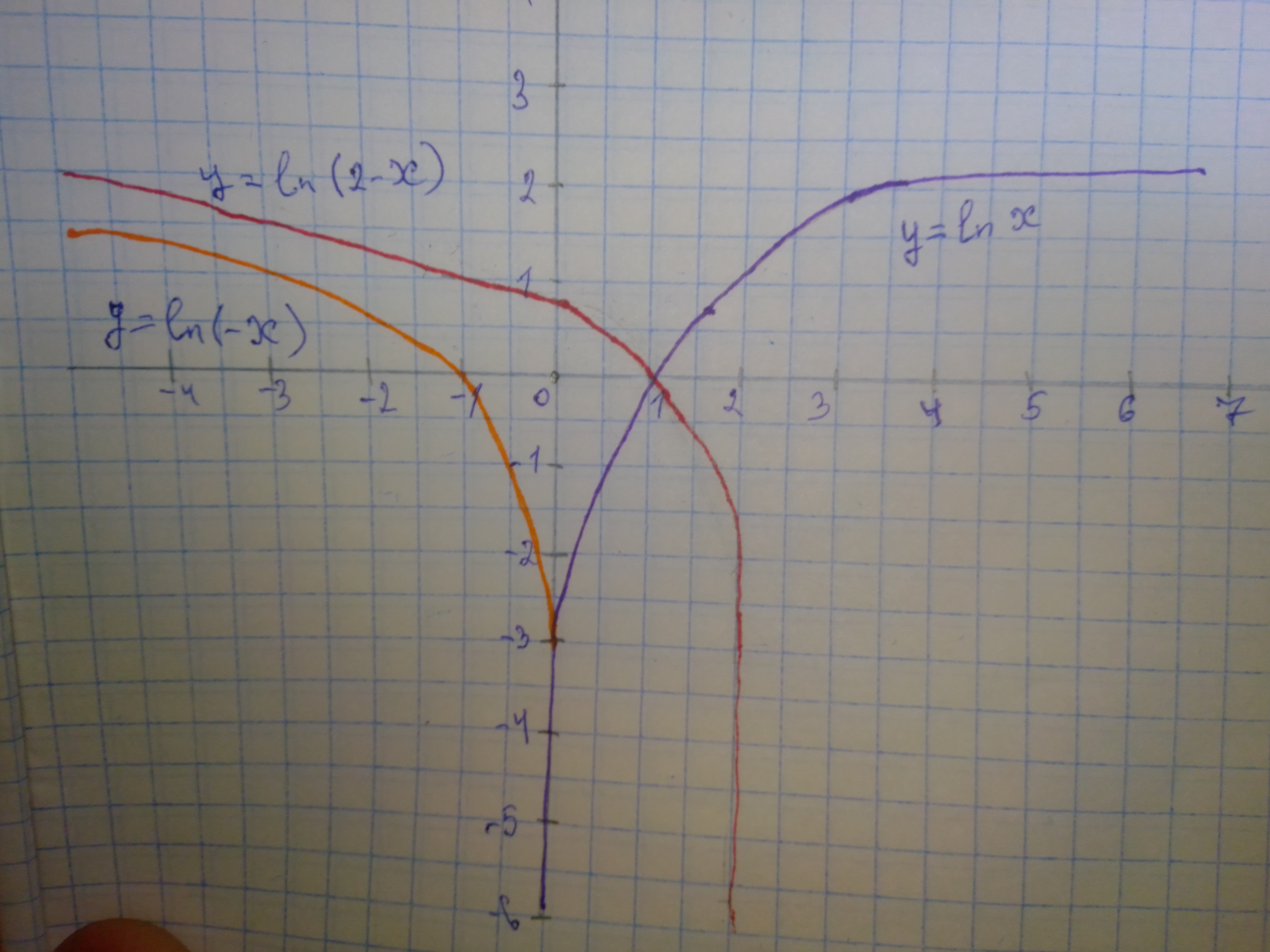Question# Begin with the graph of y = ln x and use transformations to sketch the graph of each of the given functions. y= ln (2-x)

Transformations of functions
ANSWEREDBegin with the graph of y = ln x and use transformations to sketch the graph of each of the given functions. $$\displaystyle{y}={\ln{{\left({2}-{x}\right)}}}$$Replacing x with -x in $$\displaystyle{\ln{{x}}}$$ reflects the graph about the y-axis. Replacing -x with 2 -x in $$\displaystyle{\ln{{\left(-{x}\right)}}}$$ shifts the graph 2 unit left.